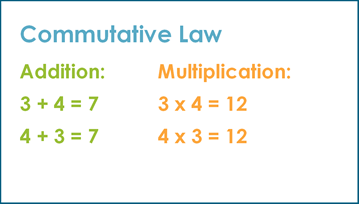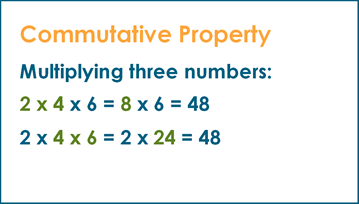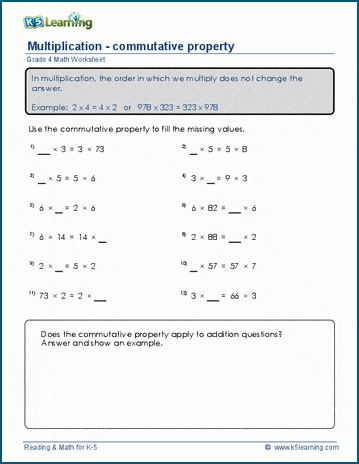# Commutative Law in Math

## What is commutative law?

The commutative law says you can get the same answer when you swap the numbers around when you add or multiply them.

3 + 4 = 7

4 + 3 = 7

In multiplication:

3 x 4 = 12

4 x 3 = 12When multiplying three or more numbers that allows students to multiply any two numbers as a first then, then multiply the product by the remaining number or numbers, no matter what the order is.

For example, when multiplying three numbers:

2 x 4 x 6 = 8 x 6 = 48

2 x 4 x 6 = 2 x 24 = 48## Commutative property worksheets

In our grade 4 math section we have a set of worksheets where students can practice multiplying multiple numbers using the commutative law.Become a Member

This content is available to members only.